Math Calculators, Lessons and Formulas

It is time to solve your math problem

mathportal.org
• Geometry
• Rectangular and square pyramid

# Rectangular and square pyramid

ans:
syntax error
C
DEL
ANS
±
(
)
÷
×
7
8
9
4
5
6
+
1
2
3
=
0
.
auto next question
calculator
•  Question 1: 1 pts The net of a paperweight is shown below. Which is closest to the lateral surface area of the paperweight?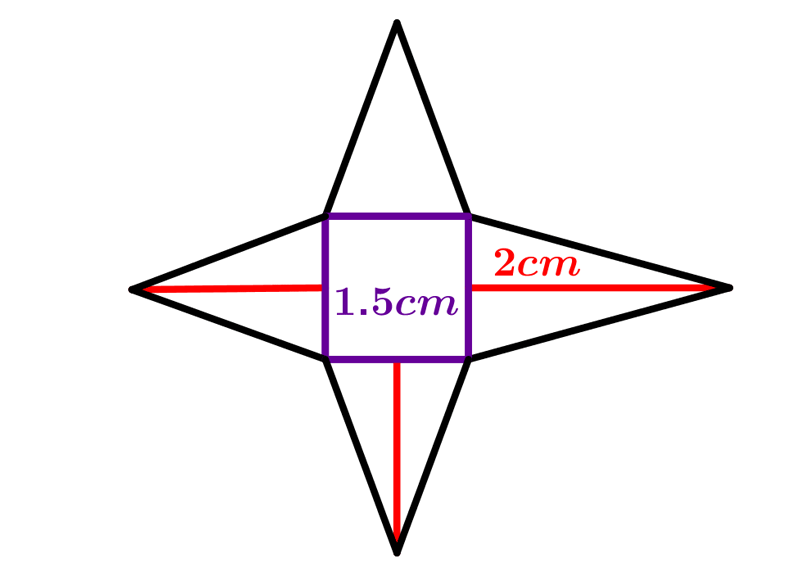$21cm^{2}$ $18cm^{2}$ $9cm^{2}$ $6cm^{32}$
•  Question 2: 1 pts The following expression can be used to find the surface area of the pyramid shown on the picture. $$A=4^{2}+4\cdot \dfrac{4\cdot 8}{2}=16+64=80cm^{2}$$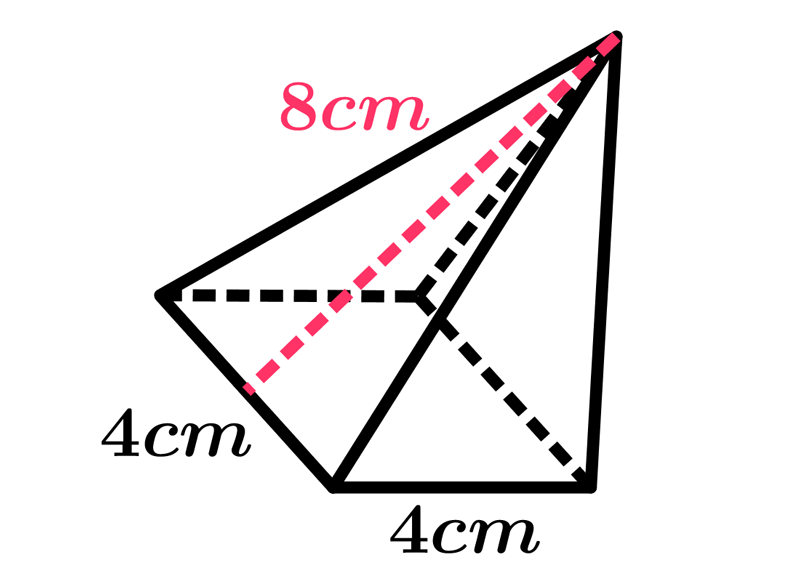•  Question 3: 1 pts The following expression can be used to find the volume of the pyramid shown on the picture. $$V=\left(5^{2}\cdot 9\right)cm^{3}=225cm^{3}$$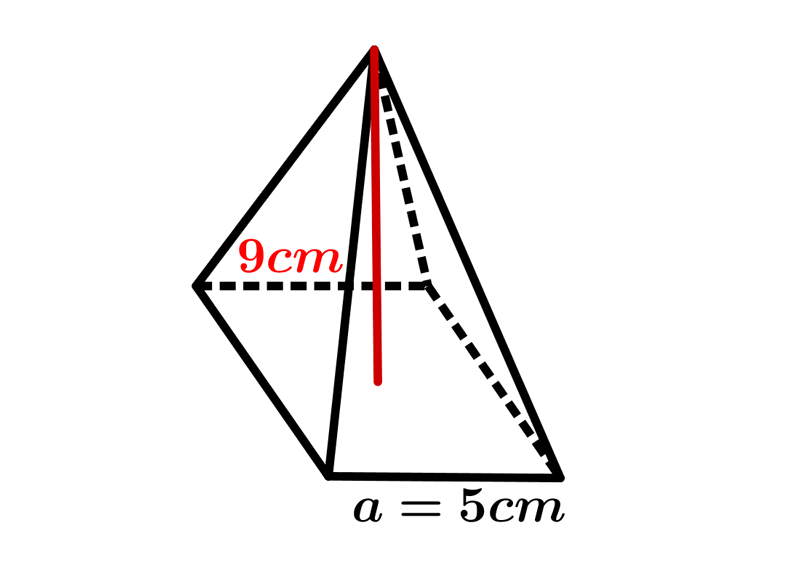•  Question 4: 1 pts The following expression can be used to find the surface area of the pyramid shown on the picture. $$A= \left(30^{2}+2\cdot 30\cdot 17 \right)cm^{2}$$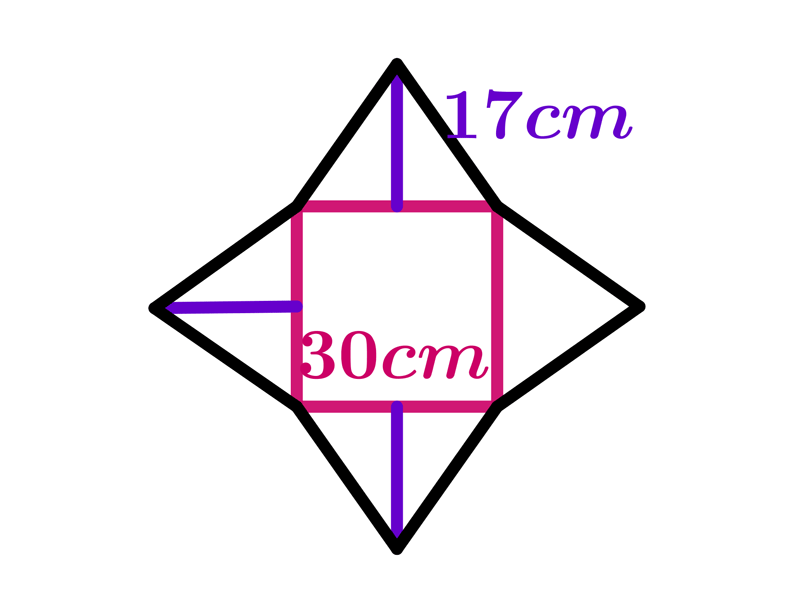•  Question 5: 2 pts Find the volume of the pyramid shown on the picture.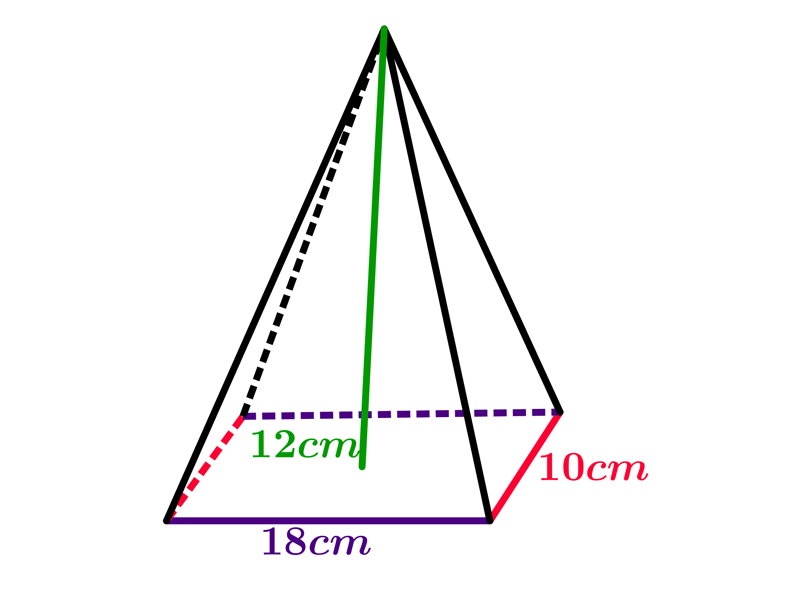$V=\left(\dfrac{1}{2}\cdot10\cdot 18\cdot 12\right) cm^{3}$ $V=\left(10\cdot 18\cdot 12\right) cm^{3}$ $V=\left(\dfrac{1}{3}\cdot10\cdot 18\cdot 12\right) cm^{3}$ $V=\left(\dfrac{1}{3}\cdot10\cdot 18\cdot 6\right) cm^{3}$
•  Question 6: 2 pts The diagonal of the base of the regular rectangular pyramid is $8\sqrt{2} cm,$ and the surface area of one lateral side is $20$ square centimeters. Find the surface area of the pyramid.
 $A=144 cm^{2}$ $A=169 cm^{2}$ $A=196 cm^{2}$ $A=225 cm^{2}$
•  Question 7: 2 pts The surface area of the base of regular square pyramid is $144$ square centimeters, and the sum of the length of base edge and lateral edge is $22 cm.$ Find the surface area od that pyramid.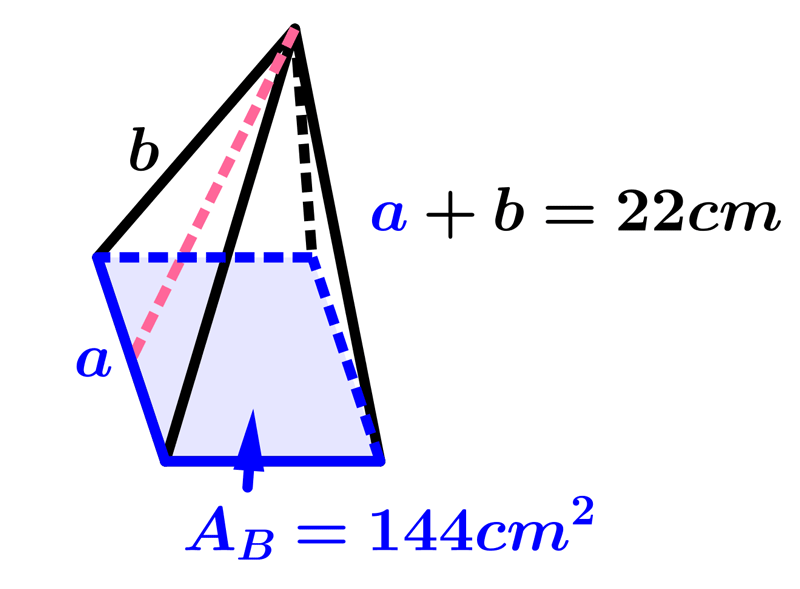$A=$
•  Question 8: 2 pts Find the height of the rectangular pyramid shown on the picture.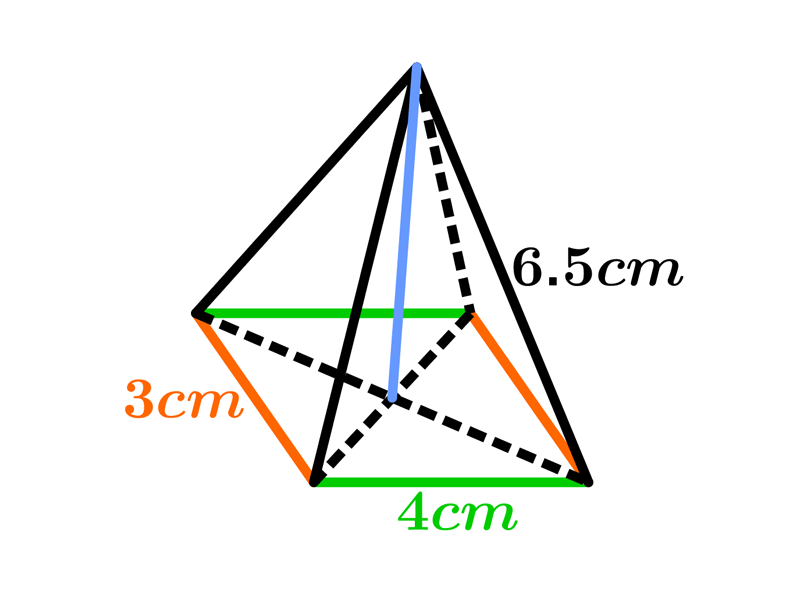Height$=$
•  Question 9: 3 pts Find the slant height of the square pyramid shown on the picture.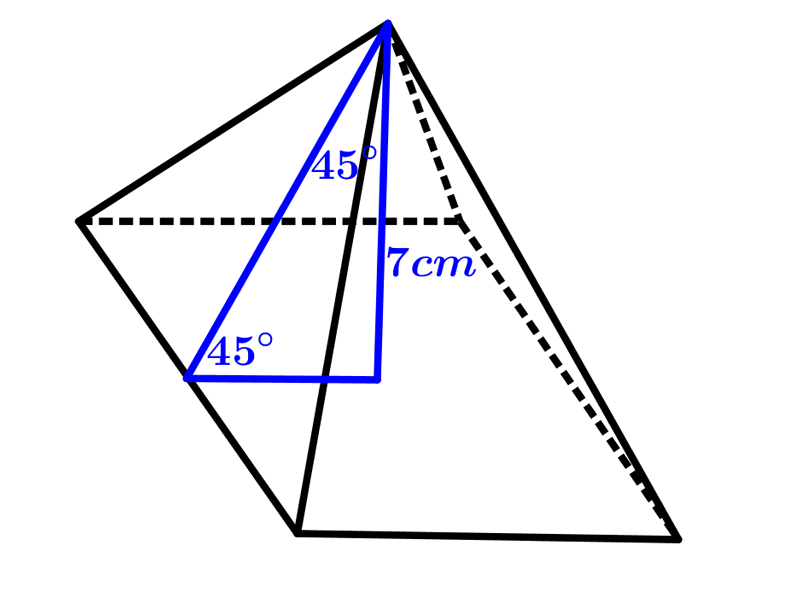$7\sqrt{2}cm$ $3\sqrt{3}cm$ $6\sqrt{3}cm$ $9\sqrt{2}cm$
•  Question 10: 3 pts The total surface area of the frustum will be $$A=32^{2}+16^{2}+4\cdot \left(\dfrac{(16+32)}{2}\cdot 10\right)cm^{2}$$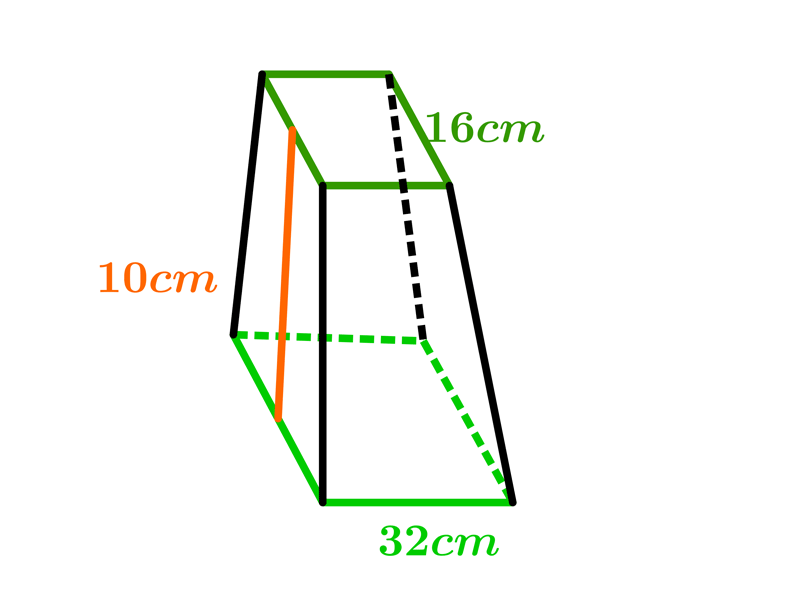•  Question 11: 3 pts A regular pyramid has a height of $11 cm$ and a square base. If the volume of the pyramid is $528$ cubic centimeters, how many centimeters are in the length of one side of its base?
 $5cm$ $8cm$ $12cm$ $14cm$
•  Question 12: 3 pts Tim has a rectangular prism with a length of $10$ centimeters, a width of $2$ centimeters and an unknown height. He need to built another rectangular prism with a length of $5$ cm and the same height as the orign prism. The volume of this two prism will be the same. Find the width, in centimeters, of the new prism.
 $4cm$ $8cm$ $10cm$ $12cm$